# Types of Elasticity

## What is elasticity?

Elasticity is a measure of the responsiveness of a variable when other variable changes. It is the proportional change of the value in one variable relative to the proportional change in the value of another variable.

We can measure the elasticity of the demand and the elasticity of the supply.

## Elasticity of Demand

When we calculate the elasticity of demand, we are measuring the relative change in the total amount of goods or services that are demanded by the market or by an individual. The quantity demanded depends on several factors. Some of the more important factors are the price of the good or service, the price of other goods and services, the income of the population or person and the preferences of the consumers.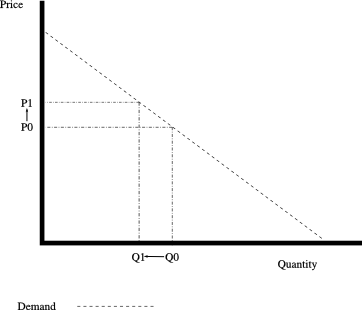Movement along the demand: when the price increases, the quantity demanded decreases

## Elasticity of Supply

When we calculate the elasticity of supply, we are measuring the relative change in the the total amount of goods or services that one or several firms supply. The quantity supplied depends on several factors. Some of the more important factors are the price of the good or service, the cost of the input and the technology of production.Movement along the supply: when the price increases, the quantity supplied decreases

As we have mentioned, the demand depends on several factors. We can calculate the elasticity of demand according to each one of these inputs.

• If we calculate the elasticity of demand according to the price of the good, we are calculating the price elasticity of demand.
• If we calculate the elasticity according to the price of other goods, we are calculating the cross elasticity of demand.
• If we calculate the elasticity of the demand according to the income, we are calculating the income elasticity of demand.

## Price elasticity of demand

The price elasticity of demand is the proportional change in the quantity demanded, relative to the proportional change in the price of the good.

Price elasticity of demand = Percentage change in quantity demanded / percentage change in price = ΔQ/Q / ΔP/P## Cross elasticity of demand

The cross elasticity of demand is the proportional change in the quantity demanded, relative to the proportional change in the price of another good.

Cross elasticity of demand = Percentaje change in quantity demanded / percentaje change in price of another good = ΔQ1/Q1 / ΔP2/P2

Looking at the chart, the change in the price of another good shifts the demand curve to the left or to the right.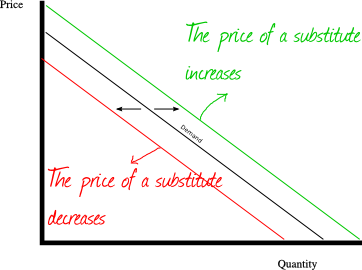If the two goods are substitutes, the cross elasticity of demand is positive.
If the two goods are complements, the cross elasticity of demand is negative.

## Income elasticity of demand

The income elasticity of demand is the proportional change in the quantity demanded, relative to the proportional change in the income.

Income elasticity of demand = Percentaje change in quantity demanded / percentaje change in the income = ΔQ/Q / ΔI/I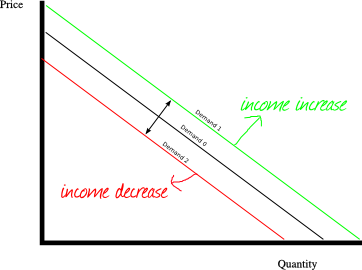## Price elasticity of supply

The price elasticity of supply is the proportional change in the quantity supplied, relative to the proportional change in the price of the good.

Price elasticity of supply = Percentaje change in quantity supplied / percentaje change in price = ΔQs/Qs / ΔP/P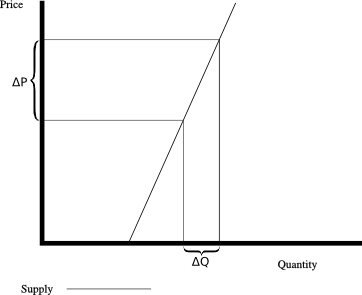## Cross elasticity of supply

The cross elasticity of supply is the proportional change in the quantity supplied, relative to the proportional change in the price of another good.

Cross elasticity of supply = Percentaje change in quantity supplied / percentaje change in the price of another good = ΔQs1/Qs1 / ΔP2/P2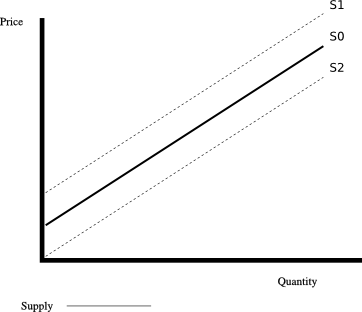Complements in production goods are goods that must be produced together. If the price of a complement in production good increases (let’s call it “good B”), then the quantity produced of B usually increases. As a result, the supply curve of the good we are analyzing (let’s call it “good A”), shift to the right. Thus, the cross elasticity of complements in production goods is positive.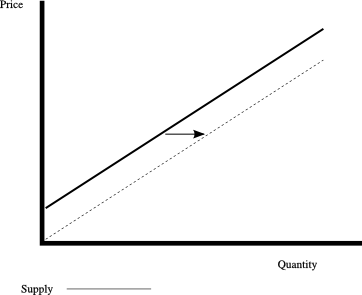Substitutes in production : goods that use the same resources for production. Producing more of one good, requires producing less of the other good. If the good B is a substitute in production of A, and the price of B increases, then the supply of the good A shifts to the left. Thus, the cross elasticity of substitutes in production goods is negative.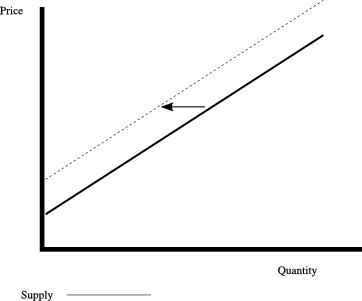Up to here, we have pointed out different types of elasticity according to the function we are analyzing, and according to the inputs we are considering. Now we will see how the supply and the demand can be classified according to the value of the elasticity.

## Elasticity of Demand

### Perfect elastic demand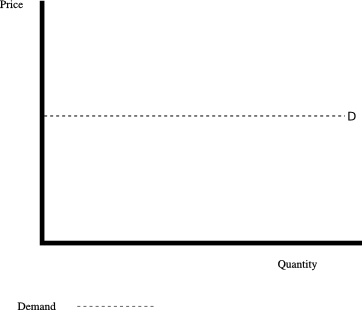Perfect Elastic Demand: The elasticity tends towards -∞.

### Relatively elastic demand, unitary elasticity demand and relatively inelastic demand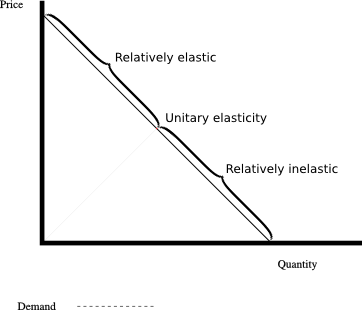Relatively elastic demand: The elasticity is between -1 and -∞
Unitary elasticity demand: The elasticity is -1
Relatively inelastic demand: The elasticity is between 0 and -1.

### Perfect inelastic demand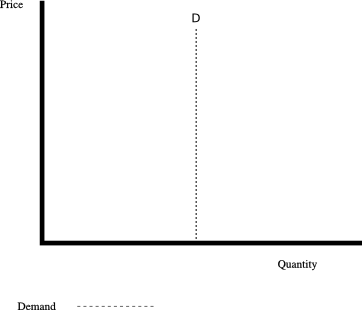A perfect inelastic demand has an elasticity of 0.

## Perfect elastic supply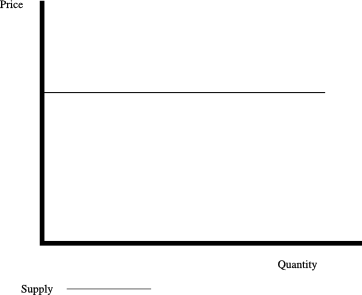An horizontal supply is a perfect elastic supply and has an elasticity that tends towards ∞

## Relatively elastic supply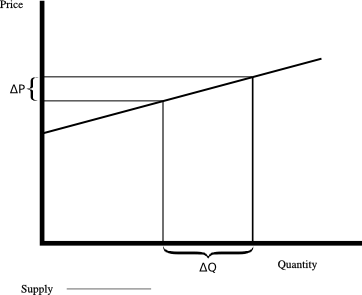A relatively elastic supply has an elasticity bigger than 1

## Supply with unitary elasticity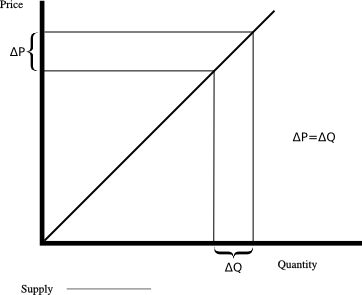An unitary elasticity supply has an elasticity of 1

## Relatively inelastic supply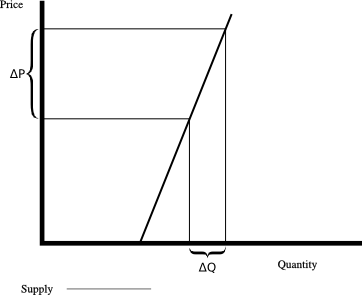A relatively inelastic supply has an elasticity of less than 1

## Perfect inelastic supply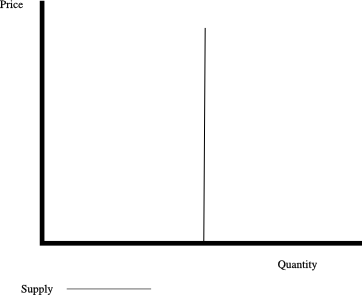A perfect inelastic supply has an elasticity of 0